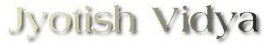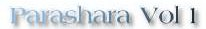Home Articles House Significations Significations of Planets Online Library Download Books Healing Power of Gems & Mantras Medical Astrology Astrologer's Directory Horoscopes Holy Tradition (Parampara) Biblical Astrology (Parampara) Mahamrtyunjaya Gayatri The Process of Karma Recommended Links Visitors Comments Recommend ReadingChapter 26.

Evaluation of Planetary Aspects

1. O Glorious, it is said that aspects (of planets) and strengths are to be known in deciding the effects. How many kinds are these? Please clarify my doubts.

2-5. PLANETARY ASPECTS:
O Brahmin, I have earlier stated aspects based on signs. The other kind is between planets which I detail below: 3rd and 10th, 5th and 9th, 4th and 8th, and lastly 7th - on these places the aspects increase gradually in slabs of quarters, i.e. 1/4, 1/2, 3/4, and full. The effects (due to such aspects) will also be proportionate. All planets aspect the 7th fully. Saturn, Jupiter, and Mars have special aspects respectively on 3rd and 10th, 5th and 9th, and 4th and 8th. The ancient preceptors have explained these which are ordinary (arising by mere sign positions). By subtle mathmatical calculations, these aspects will have to be clearly understood as under.

6-8. ASPECTUAL EVALUATIONS:
Deduct the longitude of the aspected planet (or house) from that of the aspecting planet. If the sum exceeds six signs (or 180 degrees) deduct the sum again from 10 signs ( or 300 degrees). Convert the latest sum into degress and divide by two. The resultant product is Drishti Kona or aspectual angle. If the difference (between aspector and aspected) is in excess of 5 signs, ignore signs and multiply the degrees etc. by 2 which is the aspectual value. If the difference is in excess of 4 signs, deduct it from 5 signs (or 150 degrees) and the resultant degree etc. become Drishti value. If the difference is in excess of 3 signs deduct it from 4 signs (or 120 degrees) and halve the product to be increased by 30 to get Drishti value. If the difference is above 2 signs ignore signs and add 15 to the degrees etc. to get Drishti value. If it is in excess of one sign, ignore Rasis and divide the degrees etc. by 2 to get Drishti value.

9-10. SPECIAL CONSIDERATION FOR SATURN'S ASPECTS:
O Brahmin, if Saturn is the aspecting planet find out the difference between him and the aspected planet; if the sum is above 1 sign, multiply the degrees etc, (ignoring sign) by 2 to get Drishti value. If the sum is above nine signs, the degrees to elapse be doubled to get aspectual value. If the sum is above 2 signs, the degrees etc. (in excess of 2 signs) be halved and deducted from 60. If the sum exceeds 8 signs, add to the degrees etc. a figure of 30 to get Drishti value. In other cases, the sums be process as explained earlier.

11. SPECIAL CONSIDERATION FOR MAR'S ASPECTS:
Deduct the longitude of Mars from that of the planet aspected (by Mars). If the sum is 3 Rasis & c or 7 Rasis & c, the degrees etc. (after ignoring Rasis) be reduced from 60. If it is above 2 rasis, the degrees etc. be increased by half of it (i.e. add 50%) and superadd 15. If the sum is 6 signs, one Rupa is the value.

12. SPECIAL CONSIDERATION FOR JUPITER'S ASPECTS:
Deduct the longitude of Jupiter from that of the planet aspected by him. If the resultant sum is 3 Rasis & c or 7 rasis & c, halve the degrees etc. (ignoring Rasis) and increase it by 45. If the sum is 4 Rasis & c or 8 Rasis & c, the degrees etc. ( ignoring Rasis) be subtracted from 60. This will be the aspectual value. The sum being in conformity with others than these be treated as stated earlier.